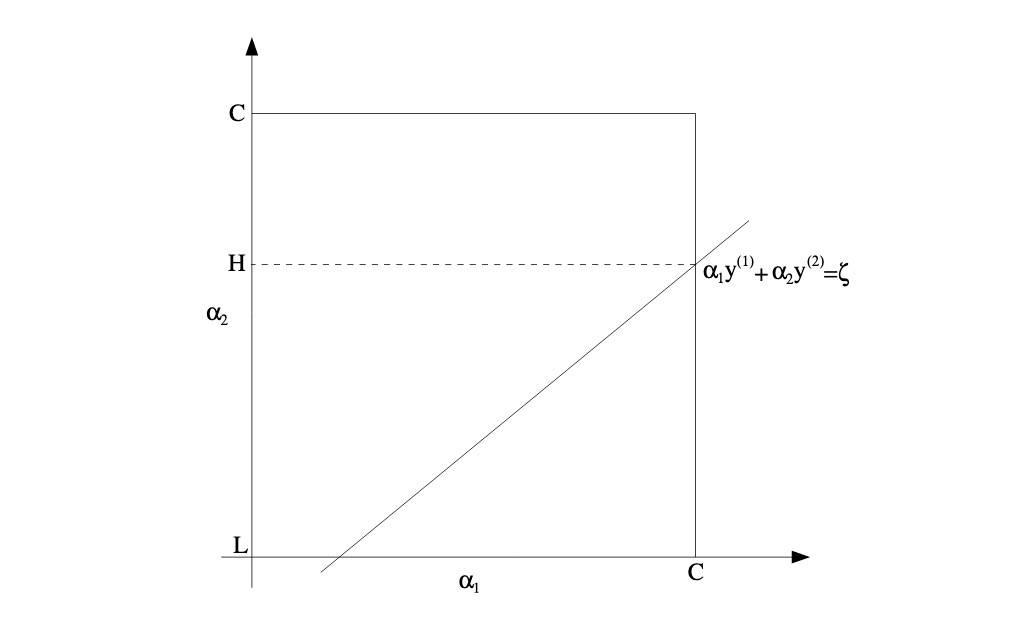# 核方法 和 SMO

## 核方法

• 感知机 PLA：大于一层隐层 + 非线性变换可以拟合任意曲线。
• 非线性可分，经过一个非线性转换将原空间的数据映射到新空间，变为线性可分（高维比低维更易线性可分）。核技巧属于这样的方法。

### 正定核

k 为对称函数。如果 K 满足以下性质：

• 对称性：K(x,z) = K(z,x)
• 正定性：任取 N 个元素，x, x2, ..., xn ∈ X，对应的 Gram 矩阵是半正定的

【希尔伯特空间】：完备的、可能是无限维的、被赋予内积的线性空间。

• 线性空间：向量空间（满足加法和数乘）
• 完备：可理解为对极限操作封闭（存在极限且极限也属于 Hilbert 空间）
• 内积，满足三个特征：
• 对称性：<f, g> = <g, f>, f,g ∈ H
• 正定性：<f, f> ≥ 0, "=" <=> f=0
• 线性：<r1f1 + r2f2, g> = r1<f, g> + r2<f2, g>

### 算法

• 输入：数据集 T = {(x1,y1), (x2, y2), ..., (xn, yn)}，其中 xi ∈ R^n, yi ∈ {+1, -1} , i=1,2,...,N
• 输出：分类决策函数

• 选择适当的核函数 K 和 惩罚参数 C，构造并求解最优化问题：

得到最优解 α* = (α1*, α2*, ..., αN*)^T

• 根据 KKT 计算 w*，并选择 α* 的一个分量 0 < αj* < C 计算 b*:

• 构造决策函数：

## SMO

SMO（Sequential minimal optimization）是一种启发式算法，主要用于解决训练样本很大时，高效地实现支持向量机的学习问题，具体看就是要解决式（1）的凸二次规划对偶问题。

Coordinate ascent 的解决思路如下：

SMO 的基本思路如下：

• 如果所有变量的解都满足 KKT 条件，那么这个解就是问题的最优解（KKT 是该最优化问题的充要条件）。
• 否则选择两个变量，固定其他变量，针对这两个变量构建一个二次规划问题。

SMO 包括两个部分：求解两个变量二次规划的解析方法和选择变量的启发式方法。

### 两个变量二次规划求解$\zeta = \alpha_1^{old} y_1 + \alpha_2^{old} y_2$ 以及 v 代入得：

φ 是输入空间到特征空间的映射。

### 变量选择

• 遍历在间隔边界上的点，依次将其对应的变量作为 α2，直到目标函数有足够的下降
• 如果找不到合适的 α2，就遍历数据集
• 如果还是找不到就放弃第一个 α1，重新选择一个

### 算法

• 输入：数据集 T = {(x1,y1), (x2, y2), ..., (xn, yn}，其中 xi ∈ R^n, yi ∈ {+1, -1} , i=1,2,...,N，精度 ε
• 输出：近似解 α

• 取初始值 α0 = 0，令 k = 0

• 选取优化变量 $\alpha_1^k, \alpha_2^k$，解析求解最优化问题（2），得到最优解 $\alpha_1^{k+1}, \alpha_2^{k+1}$，更新 α 为 $\alpha^{k+1}$

• 如果在精度 ε 内满足停机条件：

则转下一步，否则令 k=k+1，回到第二步

• 取近似解 $\hat{\alpha} = \alpha^{k+1}$

### 实现

• 首先是初始化参数，主要包括 α，b 和 E（也就是 error）
• 接下来就是初始化 α1，根据 SMO 算法，首先选择满足条件 0<αi<C 的样本点，即间隔边界上的支持向量点，检验它们是否满足 KKT 条件。如果这些点都满足，则遍历整个数据集，检验它们是否满足。
• 找到 α1 后，可以根据 E 的差距最大选择 α2，进而可以求得新的 α，b，E 和支持向量。如果 α2 不能使目标函数有足够的下降，则根据启发式规则重新选择 α2，即：
• 遍历在间隔边界上的点，依次作为 α2 直到目标函数有足够的下降
• 再不行就遍历数据集
• 还是不行就放弃第一个 α1，重新选择一个
• 直到计算完所有 α 或达到最大迭代次数为止。